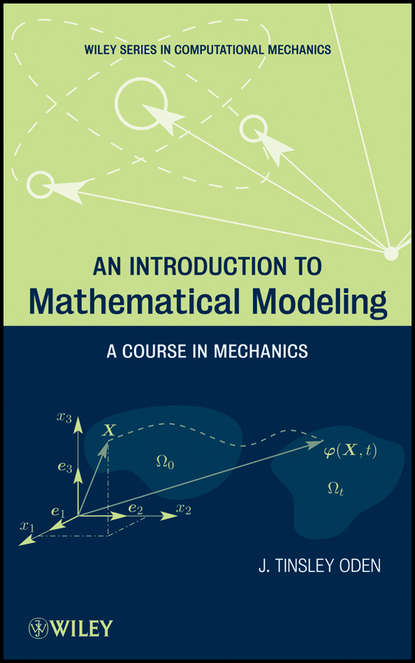Последний отзыв
Клева книга! Читала взахлеб! Очень интересно!Оценить:Рейтинг: 0

# An Introduction to Mathematical Modeling. A Course in Mechanics

Автор
Жанр
Год написания книги
2018
A modern approach to mathematical modeling, featuring unique applications from the field of mechanics An Introduction to Mathematical Modeling: A Course in Mechanics is designed to survey the mathematical models that form the foundations of modern science and incorporates examples that illustrate how the most successful models arise from basic principles in modern and classical mathematical physics. Written by a world authority on mathematical theory and computational mechanics, the book presents an account of continuum mechanics, electromagnetic field theory, quantum mechanics, and statistical mechanics for readers with varied backgrounds in engineering, computer science, mathematics, and physics. The author streamlines a comprehensive understanding of the topic in three clearly organized sections: Nonlinear Continuum Mechanics introduces kinematics as well as force and stress in deformable bodies; mass and momentum; balance of linear and angular momentum; conservation of energy; and constitutive equations Electromagnetic Field Theory and Quantum Mechanics contains a brief account of electromagnetic wave theory and Maxwell's equations as well as an introductory account of quantum mechanics with related topics including ab initio methods and Spin and Pauli's principles Statistical Mechanics presents an introduction to statistical mechanics of systems in thermodynamic equilibrium as well as continuum mechanics, quantum mechanics, and molecular dynamics Each part of the book concludes with exercise sets that allow readers to test their understanding of the presented material. Key theorems and fundamental equations are highlighted throughout, and an extensive bibliography outlines resources for further study. Extensively class-tested to ensure an accessible presentation, An Introduction to Mathematical Modeling is an excellent book for courses on introductory mathematical modeling and statistical mechanics at the upper-undergraduate and graduate levels. The book also serves as a valuable reference for professionals working in the areas of modeling and simulation, physics, and computational engineering.

## Отзывы

список сообщений пуст